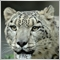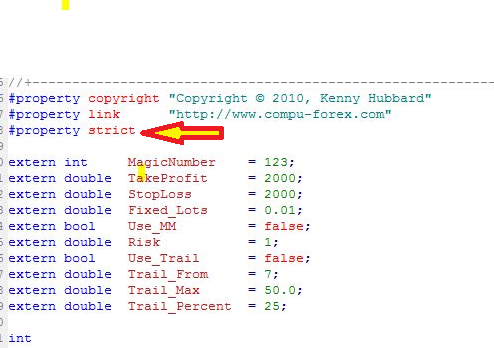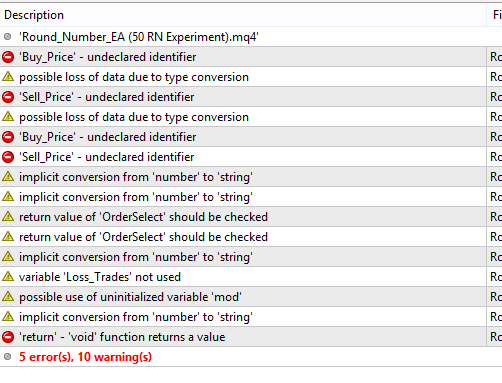# Continue to place pending orders irrespective of whether there are already open orders.22

I would be most grateful if somebody could amend (or advise me what to amend) the EA below for me please. Its is a simple EA that opens a pending buy stop and sell stop at round numbers (every 100 pips in this case). The issue I have with it is that when price moves towards to next round number, the EA does not place any more pending orders (buy stops and sell stops) unless the first trades have been closed (target hit, stop loss hit or cancelled). I would like it to continue to pending orders as price moves to the next round number irrespective of whether there are already open trades at the previous round number. The main code of the EA is copied below. Many thanks!

```extern int     MagicNumber    = 123;
extern double  TakeProfit     = 100;
extern double  StopLoss       = 160;
extern double  Fixed_Lots     = 0.1;
extern bool    Use_MM         = true;
extern double  Risk           = 1;
extern bool    Use_Trail      = false;
extern double  Trail_From     = 7;
extern double  Trail_Max      = 50.0;
extern double  Trail_Percent  = 25;

int
LotDigits = 1,
Slippage = 3,
D_Factor = 1,
Repeats = 3;
double
Pip,
Stop_Level;
string
Order_Cmt;
//+------------------------------------------------------------------+
//| expert initialization function                                   |
//+------------------------------------------------------------------+
int init()
{
Pip = Point;
if(Digits==3||Digits==5){Pip*=10;}
if(Digits==4||Digits==5){D_Factor=10;}
Slippage    *= D_Factor;
TakeProfit  *= Pip;
StopLoss    *= Pip;
Trail_From  *= Pip;
Trail_Max   *= Pip;
Stop_Level = MathMax(0.2 * Pip, MarketInfo(OrderSymbol(),MODE_STOPLEVEL));
if (MarketInfo(Symbol(),MODE_LOTSTEP) == 0.01)LotDigits = 2;
Order_Cmt = WindowExpertName();
//----
return(0);
}
//+------------------------------------------------------------------+
//| expert deinitialization function                                 |
//+------------------------------------------------------------------+
int deinit()
{
//----

//----
return(0);
}
//+------------------------------------------------------------------+
//| expert start function                                            |
//+------------------------------------------------------------------+
int start()
{
int
lRepeats = Repeats;
static bool
OCO_Done;
int
if(!My_Orders){
double
My_Price_Raw = ((Ask+Bid)/2) * (10 * D_Factor),
My_Price = MathRound(My_Price_Raw);
if(My_Price>My_Price_Raw){
double
Sell_Price = NormalizeDouble((My_Price-1)/(10*D_Factor),Digits);
double
P_Diff_Sell = MathAbs(Bid-Sell_Price);

}
else{
Sell_Price = NormalizeDouble(My_Price/(10*D_Factor),Digits);
}
OCO_Done = false;
}
if(!OCO_Done){
if(My_Orders>0){
if(Order_Taken())OCO_Done = Pending_Delete();
}
}
if(Use_Trail)Trail_Stop();
//----
return(0);
}
//+------------------------------------------------------------------+
{
double
Lots = NormalizeDouble(Fixed_Lots,LotDigits);
if(Use_MM)Lots = NormalizeDouble(Get_Lots(),LotDigits);
int
result = 0;
double
Sell_SL = NormalizeDouble(S_Price + StopLoss,Digits),
Sell_TP = NormalizeDouble(S_Price - TakeProfit,Digits);
if(B_Ticket<0)Print("Buy ordersend error - error = " + GetLastError());
if(B_Ticket>0)result++;
int S_Ticket = OrderSend(Symbol(),OP_SELLSTOP,Lots,S_Price,30,Sell_SL,Sell_TP,Order_Cmt,MagicNumber,0,CLR_NONE);
if(S_Ticket<0)Print("Sell ordersend error - error = " + GetLastError());
if(S_Ticket>0)result++;
if(result==2)return(true);
Pending_Delete();
return(false);
}
//+------------------------------------------------------------------+
{
int
cnt=0;
for(int i=OrdersTotal()-1;i>=0;i--){
OrderSelect(i,SELECT_BY_POS);
if(OrderMagicNumber()==MagicNumber){
if(OrderSymbol()==Symbol())cnt++;
}
}
return(cnt);
}
//+------------------------------------------------------------------+
bool Order_Taken()
{
for(int i=OrdersTotal()-1;i>=0;i--){
OrderSelect(i,SELECT_BY_POS);
if(OrderMagicNumber()==MagicNumber){
if(OrderSymbol()==Symbol()){
if(OrderType()<2)return(true);
}
}
}
return(false);
}
//+------------------------------------------------------------------+
bool Pending_Delete()
{
bool
result = false;
for(int i=OrdersTotal()-1;i>=0;i--){
if(OrderSelect(i,SELECT_BY_POS)){
if(OrderMagicNumber()==MagicNumber){
if(OrderSymbol()==Symbol()){
if(OrderType()>1)result = OrderDelete(OrderTicket());
}
}
}
else Print("Error selecting order in Pending_Delete function - Error = " + GetLastError());
}
return(result);
}
//+------------------------------------------------------------------+
double Get_Lots()
{
static bool
In_Recovery = false;
static int
double
lStop = StopLoss;
lStop /= Pip;
double
Pip_Value = MarketInfo(Symbol(),MODE_TICKVALUE) * D_Factor,
Lot_Value = MarketInfo(Symbol(),MODE_LOTSIZE) * D_Factor,
Money_Loss = Risk/Lot_Value * AccountBalance(),
}
//+------------------------------------------------------------------+
void Trail_Stop()
{
bool
mod;
int
err;
double
My_Profit,
My_Trail,
My_SL;
//----
for (int i = 0; i < OrdersTotal(); i++){
if(OrderMagicNumber() == MagicNumber){
RefreshRates();
switch(OrderType()){
case OP_BUY :  My_Profit = MarketInfo(OrderSymbol(), MODE_BID) - OrderOpenPrice();
My_Trail = MathMin(My_Profit * Trail_Percent/100,Trail_Max);
My_SL = NormalizeDouble(MarketInfo(OrderSymbol(),MODE_BID)-My_Trail,Digits);
if(My_Profit > Trail_From){
if(MarketInfo(OrderSymbol(),MODE_BID) - My_SL > Stop_Level){
if(OrderStopLoss() < My_SL || OrderStopLoss() == 0) mod = OrderModify(OrderTicket(),OrderOpenPrice(),My_SL,OrderTakeProfit(),0, CLR_NONE);
}
}
break;

case OP_SELL : My_Profit = OrderOpenPrice() - MarketInfo(OrderSymbol(), MODE_ASK);
My_Trail = MathMin(My_Profit * Trail_Percent/100,Trail_Max);
if(My_Profit > Trail_From){
if(My_SL < OrderStopLoss() || OrderStopLoss() == 0) mod = OrderModify(OrderTicket(),OrderOpenPrice(),My_SL,OrderTakeProfit(),0,CLR_NONE);
}
}
break;
}
if(!mod){
err = GetLastError();
if (err > 1) Print("Error entering Trailing Stop - Error (" + err + ")");
}
}
}
else Print("Error selecting order");
}
return(0);
}
//+------------------------------------------------------------------+```882

```bool Order_Taken()
{
for(int i=OrdersTotal()-1;i>=0;i--){
OrderSelect(i,SELECT_BY_POS);
if(OrderMagicNumber()==MagicNumber){
if(OrderSymbol()==Symbol()){
if(OrderType()<2)return(true);
}
}
}
return(false);```

Copy & paste this to the top of your code and try it again

`#property strict`

Take a look at some of your return(), most don't return a value.

what bool is return(false)?

you haven't returned a value.

Doesn't this cause an error?

```void Trail_Stop()
{
bool
mod;
int
err;
double
My_Profit,
My_Trail,
My_SL;
//----
for (int i = 0; i < OrdersTotal(); i++){
if(OrderMagicNumber() == MagicNumber){
RefreshRates();
switch(OrderType()){
case OP_BUY :  My_Profit = MarketInfo(OrderSymbol(), MODE_BID) - OrderOpenPrice();
My_Trail = MathMin(My_Profit * Trail_Percent/100,Trail_Max);
My_SL = NormalizeDouble(MarketInfo(OrderSymbol(),MODE_BID)-My_Trail,Digits);
if(My_Profit > Trail_From){
if(MarketInfo(OrderSymbol(),MODE_BID) - My_SL > Stop_Level){
if(OrderStopLoss() < My_SL || OrderStopLoss() == 0) mod = OrderModify(OrderTicket(),OrderOpenPrice(),My_SL,OrderTakeProfit(),0, CLR_NONE);
}
}
break;

case OP_SELL : My_Profit = OrderOpenPrice() - MarketInfo(OrderSymbol(), MODE_ASK);
My_Trail = MathMin(My_Profit * Trail_Percent/100,Trail_Max);
if(My_Profit > Trail_From){
if(My_SL < OrderStopLoss() || OrderStopLoss() == 0) mod = OrderModify(OrderTicket(),OrderOpenPrice(),My_SL,OrderTakeProfit(),0,CLR_NONE);
}
}
break;
}
if(!mod){
err = GetLastError();
if (err > 1) Print("Error entering Trailing Stop - Error (" + err + ")");
}
}
}
else Print("Error selecting order");
}
return(0);
}
```22

Thank you for your response GrumpyDuckMan. I am actually not versed in mql4 coding (ie. I am a complete novice) and this EA was not written by me. As the EA stands, it does work and causes no errors. However, like already explained, it just opens pending orders for a pair only after a previous order for that pair has been closed. I, however, want it to open pending orders irrespective of their already being open orders. Thanks22

Hello again GrumpyDuckMan. I copied and pasted the code as your instructed,but got the following errors after I compiled it:882

Hello again,

It looks like you have some problems with your code. The errors you need to fix, but the warnings you can leave. I would fix the warning about "return values of OrderSelect should be checked"882

Hello again, try this

```//+------------------------------------------------------------------+
//|                                                      ProjectName |
//|                                       http://www.companyname.net |
//+------------------------------------------------------------------+
#property strict
extern int     MagicNumber    = 123;
extern double  TakeProfit     = 100;
extern double  StopLoss       = 160;
extern double  Fixed_Lots     = 0.1;
extern bool    Use_MM         = true;
extern double  Risk           = 1;
extern bool    Use_Trail      = false;
extern double  Trail_From     = 7;
extern double  Trail_Max      = 50.0;
extern double  Trail_Percent  = 25;

bool mod;
int err,lRepeats,My_Orders,LotDigits=1,Slippage=3,D_Factor=1,Repeats=3,B_Ticket,S_Ticket;
static bool OCO_Done;
static bool In_Recovery=false;
string Order_Cmt;
//+------------------------------------------------------------------+
//| expert initialization function                                   |
//+------------------------------------------------------------------+
int init()
{
Pip=Point;
if(Digits==3||Digits==5){Pip*=10;}
if(Digits==4||Digits==5){D_Factor=10;}
Slippage    *= D_Factor;
TakeProfit  *= Pip;
StopLoss    *= Pip;
Trail_From  *= Pip;
Trail_Max   *= Pip;
Stop_Level=MathMax(0.2*Pip,MarketInfo(OrderSymbol(),MODE_STOPLEVEL));
if(MarketInfo(Symbol(),MODE_LOTSTEP)==0.01)LotDigits=2;
Order_Cmt=WindowExpertName();
//----
return(0);
}
//+------------------------------------------------------------------+
//| expert deinitialization function                                 |
//+------------------------------------------------------------------+
int deinit()
{
//----

//----
return(0);
}
//+------------------------------------------------------------------+
//| expert start function                                            |
//+------------------------------------------------------------------+
int start()
{
lRepeats=Repeats;

if(!My_Orders)
{

if(My_Price>My_Price_Raw)
{

Sell_Price=NormalizeDouble((My_Price-1)/(10*D_Factor),Digits);

return(0);
return(0);
}
else
{
Sell_Price=NormalizeDouble(My_Price/(10*D_Factor),Digits);
return(0);
return(0);
}
OCO_Done=false;
}
if(!OCO_Done)
{
if(My_Orders>0)
{
if(Order_Taken())OCO_Done=Pending_Delete();
}
}
if(Use_Trail)Trail_Stop();
//----
return(0);
}
//+------------------------------------------------------------------+
{
Lots=NormalizeDouble(Fixed_Lots,LotDigits);

if(Use_MM)Lots=NormalizeDouble(Get_Lots(),LotDigits);
int
result=0;
Sell_SL= NormalizeDouble(S_Price+StopLoss,Digits);
Sell_TP=NormalizeDouble(S_Price - TakeProfit,Digits);
if(B_Ticket>0)result++;
S_Ticket=OrderSend(Symbol(),OP_SELLSTOP,Lots,S_Price,30,Sell_SL,Sell_TP,Order_Cmt,MagicNumber,0,CLR_NONE);
if(S_Ticket<0)Alert("Sell ordersend error - error = "+GetLastError());
if(S_Ticket>0)result++;
if(result==2)return(true);
Pending_Delete();
return(false);
}
//+------------------------------------------------------------------+
{
int cnt=0;
for(int i=OrdersTotal()-1;i>=0;i--)
{
double T=OrderSelect(i,SELECT_BY_POS);
if(OrderMagicNumber()==MagicNumber)
{
if(OrderSymbol()==Symbol())cnt++;
}
}
return(cnt);
}
//+------------------------------------------------------------------+
bool Order_Taken()
{
for(int i=OrdersTotal()-1;i>=0;i--)
{
double T=OrderSelect(i,SELECT_BY_POS);
if(OrderMagicNumber()==MagicNumber)
{
if(OrderSymbol()==Symbol())
{
if(OrderType()<2)return(true);
}
}
}
return(false);
}
//+------------------------------------------------------------------+
bool Pending_Delete()
{
bool result = false;
for(int i=OrdersTotal()-1;i>=0;i--)
{
if(OrderSelect(i,SELECT_BY_POS))
{
if(OrderMagicNumber()==MagicNumber)
{
if(OrderSymbol()==Symbol())
{
if(OrderType()>1)result=OrderDelete(OrderTicket());
}
}
}
else Print("Error selecting order in Pending_Delete function - Error = "+GetLastError());
}
return(result);
}
//+------------------------------------------------------------------+
double Get_Lots()
{
In_Recovery=false;

lStop=Pip;
Pip_Value *D_Factor;
Lot_Value=MarketInfo(Symbol(),MODE_LOTSIZE)*D_Factor;
Money_Loss = Risk/Lot_Value * AccountBalance();
}
//+------------------------------------------------------------------+
void Trail_Stop()
{
for(int i=0; i<OrdersTotal(); i++)
{
{
if(OrderMagicNumber()==MagicNumber)
{
RefreshRates();
switch(OrderType())
{
My_Trail = MathMin(My_Profit * Trail_Percent/100,Trail_Max);
My_SL=NormalizeDouble(MarketInfo(OrderSymbol(),MODE_BID)-My_Trail,Digits);
if(My_Profit>Trail_From)
{
if(MarketInfo(OrderSymbol(),MODE_BID)-My_SL>Stop_Level)
{
if(OrderStopLoss()<My_SL || OrderStopLoss()==0) mod=OrderModify(OrderTicket(),OrderOpenPrice(),My_SL,OrderTakeProfit(),0,CLR_NONE);
}
}
break;

My_Trail = MathMin(My_Profit * Trail_Percent/100,Trail_Max);
if(My_Profit>Trail_From)
{
{
if(My_SL<OrderStopLoss() || OrderStopLoss()==0) mod=OrderModify(OrderTicket(),OrderOpenPrice(),My_SL,OrderTakeProfit(),0,CLR_NONE);
}
}
break;
}
if(!mod)
{
err=GetLastError();
if(err>1) Alert("Error entering Trailing Stop - Error ("+err+")");
}
}
}
}
//return(0);
}
//+------------------------------------------------------------------+
```22

GrumpyDuckMan, I thank you for your assistance and I am most grateful. I have compiled and run you code above. Unfortunately, it does not do what I want either. That is to to continue to place pending orders at the next round numbers above and below the price as the price moves irrespective of there being open trades that have not been closed. Thanks882

Hello again,

I didn't fix your code to work. I just removed the errors. there are still problems with it. Maybe if you try debugging it you will see what I mean.22

Hello GrumpyDuckMan. Thank you for your response. The code works as it is currently. All I was just after was the amendment I have repeatedly mentioned - continue to open pending orders (buy stop above and sell stop below current price at round numbers) even if there are already open orders yet to be closed for the pair. Thanks882

Hello again,

you have almost identified one of your problems. Please try what I asked you to do. you will be a little surprised as to what the code is actually doing. Just add variables to watch windows in debug mode and use F11 to step through it.

I can tell you right now your code below will not work.

```bool Do_Trades(double B_Price,double S_Price)
{
Lots=NormalizeDouble(Fixed_Lots,LotDigits);

if(Use_MM)Lots=NormalizeDouble(Get_Lots(),LotDigits);
int
result=0;
Sell_SL= NormalizeDouble(S_Price+StopLoss,Digits);
Sell_TP=NormalizeDouble(S_Price - TakeProfit,Digits);